# Balls

Ping pong balls have a diameter of approximately 5.1 cm. It sold in boxes of 10 pieces: each box has a cuboid shape with a square base. The balls touch the walls of the box.

Calculate what portion of the internal volume of the box is filled with balls.

x =  52.36 %

### Step-by-step explanation: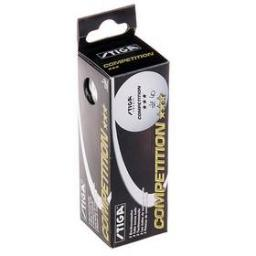Did you find an error or inaccuracy? Feel free to write us. Thank you!

Showing 1 comment:UnkownTips to related online calculators
Check out our ratio calculator.
Tip: Our volume units converter will help you with the conversion of volume units.

#### You need to know the following knowledge to solve this word math problem:

We encourage you to watch this tutorial video on this math problem:

## Related math problems and questions:

• Juice box 2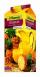Box with juice has the shape of a cuboid. Internal dimensions are 15 cm, 20 cm, and 32 cm. Suppose the box stays at the smallest base juice level reaches 4 cm below the upper base. How much internal volume of the box fills juice? How many cm below the bas
• FreezerThe freezer has the shape of a cuboid with internal dimensions of 12 cm, 10 cm, 30 cm. A layer of ice of 23 mm thick was formed on the inner walls (and on the opening) of the freezer. How many liters of water will drain if we dispose the freezer?
• PebbleThe aquarium with internal dimensions of the bottom 40 cm × 35 cm and a height of 30 cm is filled with two-thirds of water. Calculate how many millimeters the water level in the aquarium rises by dipping a pebble-shaped sphere with a diameter of 18 cm.
• AquariumThe box-shaped aquarium is 40 cm high; the bottom has dimensions of 70 cm and 50 cm. Simon wanted to create an exciting environment for the fish, so he fixed three pillars to the bottom. They all have the shape of a cuboid with a square base. The base edg
• Uboid volumeCalculate the cuboid volume if the walls are 30cm², 35cm², 42cm²
• Cuboid wallsCalculate the cuboid volume if its different walls have an area of 195cm², 135cm², and 117cm².
• Cardboard boxWe want to make a cardboard box shaped quadrangular prism with rhombic base. Rhombus has a side of 5 cm and 8 cm one diagonal long. The height of the box to be 12 cm. The box will be open at the top. How many square centimeters cardboard we need, if we ca
• Mr. Gardener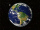Mr. Gardener wants to make wood for the balcony. Boxes. Each will have the shape of a perpendicular prism with a square base, the height is limited to 60 cm. Each container will be filled with soil by pouring the whole bag of substrate sold in a package w
• Cuboid - complicatefThree walls of the same cuboid have content 6 cm2, 10 cm2, and 15 cm2. Calculate the volume of the cuboid.
• Four prismsQuestion No. 1: The prism has the dimensions a = 2.5 cm, b = 100 mm, c = 12 cm. What is its volume? a) 3000 cm2 b) 300 cm2 c) 3000 cm3 d) 300 cm3 Question No.2: The prism base is a rhombus with a side length of 30 cm and a height of 27 cm. The height of t
• Cylinder in cube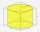Into a paper box in the shape of a cube with an edge of 10 cm is placed a can in the shape of a cylinder with a height of 10 cm and touching all the walls of the cube. What % of the volume of the cube can take up?
• Max - cone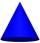From the iron bar (shape = prism) with dimensions 6.2 cm, 10 cm, 6.2 cm must be produced the greatest cone. a) Calculate cone volume. b) Calculate the waste.
• CannonballsOf the three cannonballs with a diameter of 16 cm, which landed in the castle courtyard during the battle, the castle blacksmith cast balls with a diameter of 10 cm, which fit into the cannons placed on the walls. How many cannonballs did the blacksmith c
• Paper box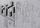Calculate the consumption of paper on the box-shaped quadrangular prism with rhombic footstall, base edge a=6 cm and the adjacent base edges forms an angle alpha = 60 °. Box height is 10 cm. How many m2 of the paper consumed 100 such boxes?
• Bottles of juice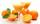How many 2-liter bottles of juice need to buy if you want to transfer juice to 50 pitchers rotary cone shape with a diameter of 24 cm and base side length of 1.5 dm.
• ContainerThe container-shaped box with internal dimensions of 3.9 m, 3.25 m and 2.6 m was completely filled with goods in the same cubic boxes. How long edge could this box have?
• Pine woodFrom a trunk of pine 6m long and 35 cm in diameter with a carved beam with a cross-section in the shape of a square so that the square had the greatest content area. Calculate the length of the sides of a square. Calculate the volume in cubic meters of lu